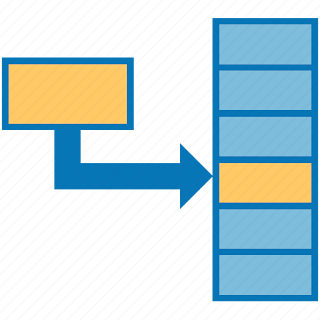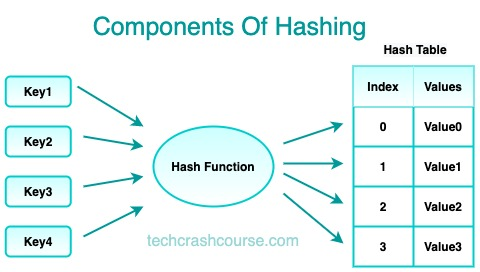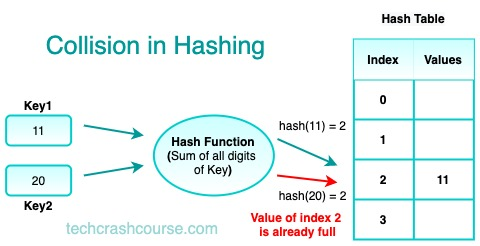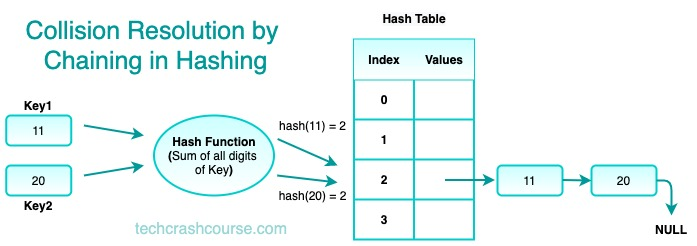# Hash Table Data Structure# Hash Table

A hash table is a data structure that is used to store and retrieve data quickly. It uses a technique called hashing to map keys to indexes in an array. The hash table consists of an array of fixed size, and each slot in the array can store a key-value pair.

The keys are used to compute an index into the array using a hash function. Once the index is computed, the value can be stored or retrieved from the corresponding slot.

Hash tables are widely used in computer science due to their fast insertion, deletion, and lookup operations. They are commonly used in applications where large amounts of data need to be stored and retrieved quickly, such as databases, caches, and compilers.## Hashing (Hash Function)

Hashing is the process of converting a key into an index that can be used to access the corresponding value in the hash table. The hash function takes a key as input and returns an index into the array. The hash function should be fast and should produce evenly distributed indices to avoid collisions.

The ideal hash function should produce a unique index for each key. However, this is not always possible, and collisions can occur when two or more keys map to the same index.

## Hash Collision

A hash collision occurs when two or more keys are mapped to the same index in the array. Hash collisions are inevitable in hash tables due to the limited size of the array and the potentially infinite number of keys that can be inserted.

To handle collisions, various collision resolution techniques can be used, such as chaining, linear probing, and quadratic probing.## Collision Resolution by Chaining

Chaining is a collision resolution technique where each slot in the hash table contains a linked list of key-value pairs. When a collision occurs, the key-value pair is added to the linked list at the corresponding slot.

To retrieve a value, the hash function is used to compute the index of the key, and then the linked list at that index is searched for the key-value pair.

Chaining is simple to implement and works well when the load factor (the ratio of the number of elements to the size of the array) is low. However, as the load factor increases, the length of the linked lists also increases, leading to a decrease in performance.### Basic Hash Function Examples

• Division Function: The hash function computes the index as the remainder of the key divided by the size of the array. This method works well when the size of the array is a prime number and the keys are uniformly distributed.

• Multiplication Function: The hash function computes the index as the product of the key and a constant factor, then takes the fractional part of the result and multiplies it by the size of the array. This method works well when the constant factor is a fractional number between 0 and 1 and the keys are uniformly distributed.

• Universal Hashing Function: The hash function uses a family of hash functions, each of which is chosen randomly from a set of hash functions. This method works well when the keys are not uniformly distributed, as it reduces the likelihood of collisions.

### Common Hash Table Operations and Their Time Complexities

The time complexity of the common operations on a Singly Linked List are as follows:
• Insertion: O(1) on average, O(n) in the worst case (when all keys map to the same index)
• Deletion: O(1) on average, O(n) in the worst case (when all keys map to the same index)
• Lookup: O(1) on average, O(n) in the worst case (when all keys map to the same index)
• Search: O(1) on average, O(n) in the worst case (when all keys map to the same index)
• Traversal: O(n)
The time complexity of hash table operations depends on the size of the array and the number of keys. The time complexity is O(1) on average when the load factor is low, but it can degrade to O(n) in the worst case when there are many collisions.

## Hash Table Implementation Program

This program uses a simple hash function that computes the sum of the ASCII values of the characters in the key. It implements the insert, get, and remove operations, as well as a print_table function for debugging purposes.

```#include <stdio.h>
#include <stdlib.h>
#include <string.h>

#define MAX_SIZE 100

typedef struct {
char* key;
int value;
} Pair;

typedef struct {
Pair* pairs[MAX_SIZE];
int size;
} HashTable;

int hash(char* key) {
int sum = 0;
for (int i = 0; i < strlen(key); i++) {
sum += key[i];
}
return sum % MAX_SIZE;
}

void insert(HashTable* table, char* key, int value) {
int index = hash(key);
Pair* pair = (Pair*) malloc(sizeof(Pair));
pair->key = key;
pair->value = value;
table->pairs[index] = pair;
table->size++;
}

int get(HashTable* table, char* key) {
int index = hash(key);
Pair* pair = table->pairs[index];
if (pair == NULL) {
return -1;
}
return pair->value;
}

void remove_key(HashTable* table, char* key) {
int index = hash(key);
Pair* pair = table->pairs[index];
if (pair != NULL) {
table->pairs[index] = NULL;
free(pair);
table->size--;
}
}

void print_table(HashTable* table) {
for (int i = 0; i < MAX_SIZE; i++) {
Pair* pair = table->pairs[i];
if (pair != NULL) {
printf("%s: %d\n", pair->key, pair->value);
}
}
}

int main() {
HashTable table = { .size = 0 };
insert(&table, "Alice", 25);
insert(&table, "Bob", 30);
insert(&table, "Charlie", 35);
insert(&table, "David", 40);
print_table(&table);
printf("Bob's age is %d\n", get(&table, "Bob"));
remove_key(&table, "Bob");
print_table(&table);
return 0;
}
```
Output
```Bob: 30
Alice: 25
David: 40
Charlie: 35
Bob's age is 30
Alice: 25
David: 40
Charlie: 35
```